Nithilaa Umasankar — Published On March 15, 2022 and Last Modified On March 17th, 2022

## Introduction

Remember when you were in elementary school and you were learning how to add and subtract numbers? Now, you can do the same with images also!

The input images can be subjected to arithmetic operations such as addition, subtraction, and bitwise operations (AND, OR, NOT, XOR). These operations can help to improve the qualities of the input photos.

In this article, you will understand the steps to perform arithmetic and bitwise operations on an image using the OpenCV Python package. Let’s get started!

## What does it mean by Arithmetic Operation on Images?

So, say we have two pixels in two separate photos that we wish to combine. How can we include them?
Let’s do so by imagining the following scenario. The first pixel has a colour intensity of (200, 0, 0), while the second has a colour intensity of (100, 0, 0). If we just add these values together, we obtain (300, 0, 0). This is exactly possible when dealing with RGB images.

So, how do we approach this problem in Python?
The solution comes with OpenCV library that has implemented cv2.add() and cv2.subtract() functions.

To perform these operations, you have to install the OpenCV Python library on your system. If you haven’t done that yet, then check out this link: link.

## Arithmetic Operations: Addition Of Images

Using the cv2.add() function, we can add two images. This simply adds up the picture pixels in the two images. One thing to keep in mind while performing this addition is that both images should be of the same depth and type, or the second image can just be a scalar value.

The syntax of this function is: cv2.add(img1, img2)

For this blog, we will consider the following two images for the addition of two images.Before moving to the arithmetic operations, you have to know how to read and display the loaded images. If you have any doubts on that, do check out our previous blog: link.

The added image from the above code snippet is as follows:This is just simple addition. We can blend the images using another function called cv2.addWeighted. This is similar to image addition, but images are given varied weights to create the illusion of blending or transparency.

The syntax of this function is: cv2.addWeighted(img1, wt1, img2, wt2, gammaValue)

Follow the below code snippet to perform weighted addition on two images.

The output of the weighted addition is as follows:Here I took two images to blend them together. The first image is given a weight of 0.6 and the second image is given 0.4. You can change the weights of the images as your wish!

## Arithmetic Operations: Subtraction of Images

Just like the addition of two images, you can subtract two images. This can be done using cv2.subtract() function. Note that the images to be subtracted have to be of the same size and depth.

The syntax of this function is: cv2.subtract(src1, src2)

The below code snippet shows how to subtract two images. I have subtracted the previously used images (the ones we used in addition).

The subtracted image is as follows:I also tried subtracting two simpler images to make it easier to understand. The two images I used are: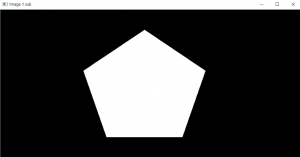The subtracted output is: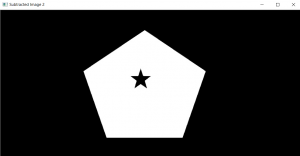Now that we have seen how to perform arithmetic operations on images, we will move on to bitwise operations on images.

## Bitwise Operations

We use bitwise operations when we need to extract only the required elements of an image.

These bitwise techniques are utilized in various computer vision applications, such as creating image masks, applying watermarks to images, and creating new images. When compared to other morphing approaches in OpenCV, these operations work on individual pixels in the image to produce more accurate results.

#### And, Or and Not operations on images

Before we begin, I’ll assume you’re familiar with the three basic bitwise operators: AND, OR, NOT.

OpenCV includes built-in functions for performing and, or, and not operations. They are bitwise and, bitwise or, and bitwise not. Consider the two black and white images below. Let us now apply these three operations to these two images and see what happens.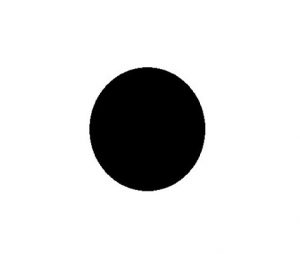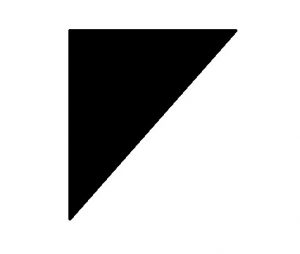The output of the above code snippet will be as follows: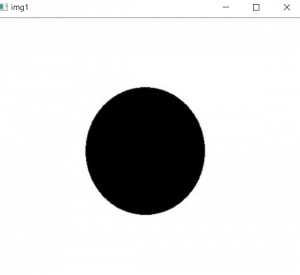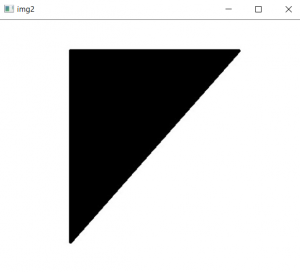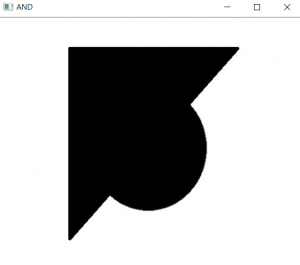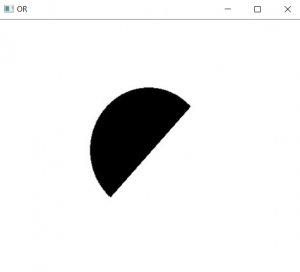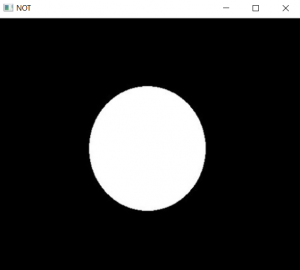I hope you’ve learned how to use OpenCV to do arithmetic and bitwise operations on images. Keep an eye out for the next OpenCV blog in this series!

Read our latest posts on our blog here.